# Keys and Rooms — Day 100 (Python)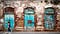Photo by Darío Martínez-Batlle on Unsplash

Today’s question is from Daily Leetcode Coding Challenge — March Edition. It is a medium-tagged question. Let us look into the problem statement.

841. Keys and Rooms

There are `N` rooms and you start in room `0`. Each room has a distinct number in `0, 1, 2, ..., N-1`, and each room may have some keys to access the next room.

Formally, each room `i` has a list of keys `rooms[i]`, and each key `rooms[i][j]` is an integer in `[0, 1, ..., N-1]` where `N = rooms.length`. A key `rooms[i][j] = v` opens the room with number `v`.

Initially, all…

# Delete Duplicate Emails — Day 99(SQL)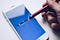Photo by Thought Catalog on Unsplash

Today’s question is a SQL question. Let us look into the problem statement.

196. Delete Duplicate Emails

Write a SQL query to delete all duplicate email entries in a table named `Person`, keeping only unique emails based on its smallest Id.

`+----+------------------+| Id | Email            |+----+------------------+| 1  | john@example.com || 2  | bob@example.com  || 3  | john@example.com |+----+------------------+Id is the primary key column for this table.`

For example, after running your query, the above `Person` table should have the following rows:

`+----+------------------+| Id | Email…`

# Generate Random Point in a Circle — Day 98(Python)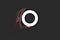Photo by Nadine Shaabana on Unsplash

Today’s question is a Math based question. It is taken from the Daily leetcode coding challenge- March edition. Let us look into the problem statement.

478. Generate Random Point in a Circle

Given the radius and x-y positions of the center of a circle, write a function `randPoint` which generates a uniform random point in the circle.

Note:

1. input and output values are in floating-point.
2. radius and x-y position of the center of the circle is passed into the class constructor.
3. a point on the circumference of the circle is considered to be in the circle.
4. `randPoint` returns a size…

# Best Time to Buy and Sell Stock with Transaction Fee — Day 97(Python)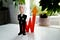Photo by Gilly on Unsplash

Today’s question is from the Daily Leetcode coding challenge — March edition. Let us look into the problem statement.

714. Best Time to Buy and Sell Stock with Transaction Fee

You are given an array `prices` where `prices[i]` is the price of a given stock on the `ith` day, and an integer `fee` representing a transaction fee.

Find the maximum profit you can achieve. You may complete as many transactions as you like, but you need to pay the transaction fee for each transaction.

Note: You may not engage in multiple transactions simultaneously (i.e., …

# Swapping Nodes in a Linked List — Day 96(Python)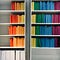Photo by Garmin Bao on Unsplash

Today’s question is taken from the Daily Leetcode Coding challenge — March edition. Let us look into the problem statement.

1721. Swapping Nodes in a Linked List

You are given the `head` of a linked list, and an integer `k`.

Return the head of the linked list after swapping the values of the `kth` node from the beginning and the `kth` node from the end (the list is 1-indexed).

Example 1:

# Check If a String Contains All Binary Codes of Size K — Day 95(Python)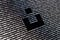Photo by Alexander Sinn on Unsplash

Today’s question is from Leetcode’s Daily coding Challenge- March Edition. Let us look into the problem statement.

1461. Check If a String Contains All Binary Codes of Size K

Given a binary string `s` and an integer `k`.

Return True if every binary code of length `k` is a substring of `s`. Otherwise, return False.

Example 1:

`Input: s = "00110110", k = 2Output: trueExplanation: The binary codes of length 2 are "00", "01", "10" and "11". They can be all found as substrings at indicies 0, 1, 3 and 2 respectively.`

Example 2:

`Input: s = "00110"…`

# First Missing Positive — Day 94(Python)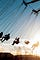Photo by Gabriel Valdez on Unsplash

Today’s question is a Hard-tagged question on Leetcode. This question is quite frequently asked in technical interviews. Let us look into the problem statement.

41. First Missing Positive

Given an unsorted integer array `nums`, find the smallest missing positive integer.

Example 1:

`Input: nums = [1,2,0]Output: 3`

Example 2:

`Input: nums = [3,4,-1,1]Output: 2`

Example 3:

`Input: nums = [7,8,9,11,12]Output: 1`

Constraints:

• `0 <= nums.length <= 300`
• `-231 <= nums[i] <= 231 - 1`

Follow up: Could you implement an algorithm that runs in `O(n)` time and uses constant extra space?

This problem is a bit tricky…

# Integer to Roman — Day 93(Python)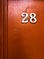Photo by Hadi Yazdi Aznaveh on Unsplash

Today’s question is a medium-tagged question in Leetcode. This question is asked in multiple coding interviews. Let us look into the problem statement.

12. Integer to Roman

Roman numerals are represented by seven different symbols: `I`, `V`, `X`, `L`, `C`, `D` and `M`.

`Symbol       ValueI             1V             5X             10L             50C             100D             500M             1000`

For example, `2` is written as `II` in Roman numeral, just two one's added together. `12` is written as `XII`, which is simply `X + II`. The number `27` is written as `XXVII`, which is `XX + V + II`.

Roman…

# Employees Earning More Than Their Managers — Day 92(SQL)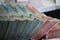Photo by Mufid Majnun on Unsplash

Today’s question is a SQL question. Let us look into the problem statement.

181. Employees Earning More Than Their Managers

The `Employee` table holds all employees including their managers. Every employee has an Id, and there is also a column for the manager Id.

`+----+-------+--------+-----------+| Id | Name  | Salary | ManagerId |+----+-------+--------+-----------+| 1  | Joe   | 70000  | 3         || 2  | Henry | 80000  | 4         || 3  | Sam   | 60000  | NULL      || 4  | Max   | 90000  | NULL      |+----+-------+--------+-----------+`

Given the `Employee` table, write a SQL query…

# Strobogrammatic Number II — Day 91(Python)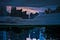Photo by jisoo kim on Unsplash

Today’s question is a medium-tagged question on leetcode. Let us look into the problem statement.

247. Strobogrammatic Number II

Given an integer `n`, return all the strobogrammatic numbers that are of length `n`. You may return the answer in any order.

A strobogrammatic number is a number that looks the same when rotated `180` degrees (looked at upside down).

Example 1:

`Input: n = 2Output: ["11","69","88","96"]`

Example 2:

`Input: n = 1Output: ["0","1","8"]`

Constraints:

• `1 <= n <= 14`

Before we start with solving this problem, let us understand what strobogrammatic numbers are. A strobogrammatic number is a…## Annamariya Tharayil

Software Engineer. Find me @ www.linkedin.com/in/annamariya-jt